﻿ Attenuation Effect in Twenty One Different Proton Dissociation Equilibriums Brought on One Rope: A Chemical Education Tool for Evaluation of p<i>K</i><SUB>a</SUB> of Proton Dissociation Equilibrium of Any Substituted Benzene (XC<SUB>6</SUB>H<SUB>5</SUB>)Publications are Open
Access in this journal
Article Versions
Export Article
• Normal Style
• MLA Style
• APA Style
• Chicago Style
Research Article
Open Access Peer-reviewed

### Attenuation Effect in Twenty One Different Proton Dissociation Equilibriums Brought on One Rope: A Chemical Education Tool for Evaluation of pKa of Proton Dissociation Equilibrium of Any Substituted Benzene (XC6H5)

World Journal of Chemical Education. 2020, 8(2), 61-66. DOI: 10.12691/wjce-8-2-1
Received January 07, 2020; Revised February 16, 2020; Accepted March 07, 2020

### Abstract

The strong empirical relation, ρ = (2.4)(2-i), between the Hammett ρ for proton dissociation of several acids and the number, “i”, of atoms between the ionizable hydrogen and the ring carbon (Andrew Williams, Free Energy Relationships in Organic and Bioorganic Chemistry, Royal Society of Chemistry, Cambridge, 2003, p. 75) is used to construct a graph for twenty one different proton dissociation equilibriums. The plot of Hammett ρ versus number of atoms i between ionizable hydrogen and the ring carbon atom is observed to be an excellent exponential-decay locus. A good average and intelligent value of Hammett ρ is obtained for the benzene dissociation equilibriums by interpolating the locus of the correlation on to Y-axis. Using this Hammett ρ value and the Hammett equation log [(Ka)X / (Ka)H] = ρσ, the pKa value can be calculated for any substituted benzene knowing the pKa value of benzene to be 43. The points for proton dissociation equilibriums of phenylethyl ammonium ions and benzyl alcohols deviated from the graph hence not included in the correlation. Possible explanations are given for deviation of these two equilibriums.

Graphical abstract

### 1. Introduction

A considerable interest on the application of attenuation effect due to methylene group was gained from our laboratory 1, 2, 3, 4. The emergence of Andrew Williams’ strong empirical relation 5 based on the existence of attenuation effect, systematically observed in the study of acid dissociation equilibriums of benzoic acids, phenyl acetic acids and phenyl propionic acids is a successful achievement. The same was extended to aromatic 1, 2, and aliphatic 3, 4 systems from our laboratory. This empirical relation 5 is further made use in the present work to bring twenty one different proton dissociation equilibrium reactions on one rope. A good average and intelligent value of Hammett ρ is obtained for the benzene dissociation equilibriums by interpolating the locus of the correlation on to Y-axis. And this study is extended to calculate pKa values using the Hammett equation log [(Ka)X / (Ka)H] = ρσ, for any substituted benzenes knowing the pKa value of unsubstituted benzene to be 43.

### 2. Methods

All calculations and curve fittings were done by Kaleida Graph software version 4.1 supplied by Synergy Software INC., Reading, PA, USA. All chemical structures were drawn using “Chemdraw” software.

### 3. Discussion

First let us see the dissociation equilibriums of different acids with increasing length of carbon chain between ionizable hydrogen and the ring carbon/nitrogen atom. Table 1 narrates twenty one different proton dissociation equilibrium reactions with Hammett ρ values, and the number of atoms between ionizable hydrogen and the ring carbon/nitrogen. The Hammett ρ values are given in the increasing order down to the table. Using the Williams’ equation 1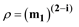(1)

where m1 is an arbitrary constant, a plot of Hammett ρ versus number of atoms between ionizable hydrogen and ring carbon is made and shown in Figure 1. From equation 1 it is clear that if i = 0, i.e. if there are no atoms between the ionizable hydrogen and the ring carbon atom, the example would be benzene itself. Then the Hammett ρ value would be m1(2 - i) = m1(2 - 0). Here, from the curve fit of the data, m1 was found to be 2.4917 (see box in the figure). Therefore the Hammett ρ value for proton dissociation equilibrium (equation 2) of benzene will be (2.4917)2 = 6.27.(2)
• Figure 1. Plot of Hammett ρ versus number of atoms (m0) between ioniable hydrogen and the ring carbon atom

And this value is very close to the average (5.6 + 6.6 = 12.2 /2 =6.1) of the two values previously reported, one for proton dissociation equilibrium of benzene1 and the other proton dissociation equilibrium of pyridinium ions 2 (equation 3). In both the cases “i”, number of(3)

atoms between ionizable hydrogen and ring carbon/ nitrogen is zero.

But the points of the equilibriums of the compounds 9 and 11 deviated from the correlation. The deviation could be due to the anomalous Hammett ρ obtained from the anomalous pKa values of phenylethyl ammonium ions 6. The anomalistic pKa values are due to the formation of the more stable zwitter ion of 4-hydroxy phenylethyl ammonium ion and even more stabilization of the 3, 4-dihydroxy phenylethyl ammonium ion due to hydrogen bonding, both are as shown below (equations 4 and 5)(4)

and(5)

The anomalous Hammett ρ obtained from the proton dissociation equilibrium of benzyl alcohols is due to: the Hammett ρ value for benzyl alcohols dissociation equilibriums should be simply 1.11 7, which is half of that of phenoxide ion equilibriums, based on the fact that the Hammett ρ value for phenyl acetic acids has become reduced to half of that of benzoic acids upon the introduction of one CH2 group between the carboxyl ate carbon and the benzene ring carbon. But the actual ρ value was found to be 1.73 8. One putative explanation of this, offered by Wiberg 9, is: In gas-phase, alkoxide ions, the negatively charged oxygen atom polarizes the C-H bonds, placing considerable negative charge at the hydrogen atoms and a positive charge at the carbon atom. The carbon has an attractive Coulombic interaction with the negatively charged oxygen, leading to a shorter C-O bond, which may lead to somewhat larger substituent effect and in turn in aqueous solution even larger. Another explanation may come from the hyperconjugation of the benzyloxide ion, which may be even further stabilized by charge delocalization onto benzene ring as it is shown below (Equation. 6) to contribute towards higher Hammett ρ value.(6)

Calculation of pKa of any substituted benzene: The Hammett ρ for the proton dissociation equilibriums (equation 2) from the present work is 6.27. And the Hammett equation is: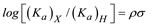(7)

Rewriting this equation in terms of pKa we get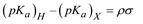(8)

(pKa)H of benzene is 43 10, Hammett ρ is 6.27 and if the (pKa)X of nitrobenzene (for X = NO2) is to be calculated, assuming the ‘the hydrogen” para to nitro group in benzene ring is to be deprotonated as shown in equation 9, then σ for 4-NO2 substituent has to be taken as 0.78 7.(9)

Substituting these values in equation 8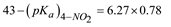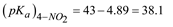Therefore the pKa of nitrobenzene with deprotonation at para position is 38.1. Similarly for any substituted benzene the pKa values could be calculated.

### References

  Jagannadham V 2009 The attenuation effect through methylene group. Bulgarian Chem. Commns. 41 50 In article View Article  Sanjeev R, Jagannadham V and Veda Vrath R 2014 Attenuation effect through methylene group: Part II, Bulgarian Chem. Commns. 46 375 In article  Sanjeev R and Jagannadham V 2017 Estimation of Taft ρ* of dissociation equilibriums of methanium ions RCH4+ the hydrocarbon super acids: a chemical education practice in physical-organic chemistry class-room, Current Physical Chemistry 7 218 In article View Article  Sanjeev Rachuru, Jagannadham Vandanapu and Sreedhar Pandiri, Tetrahedral Nature Determines the Stability of Reactive Intermediates: A Chemical Education Perspective, Oriental J. Chemistry, 2019 (in press) In article View Article  A. Williams, Free Energy Relationships in Organic and Bioorganic Chemistry, Royal Society of Chemistry, Cambridge, 2003, p. 75 In article  Hammett ρ is calculated from the plot of pKa versus Hammett σ values of only 4-H, 4-OH and 3-OH substituted phenyl ethyl ammonium ions. pKa values are from Murray M. Tuckerman, J. Richard Mayer and Frederick C. Nachod, J. Am. Chem. Soc., 1959, 81, 92 In article View Article  H. H. Jaffe, Chem. Rev, 53, 191 (1953) In article View Article  The ρ value for this equilibrium is an estimate based on the correlation of gas-phase ∆Hacid values9 of different oxygen containing acids and is done as follows: The Hammett ρ value for phenol dissociation equilibriums in aqueous solution is 2.217. The gas-phase ρ value for this same equilibrium is 1.289. Hence the ratio (ρaq/ρgas) is 1.73. The Hammett ρ value from the correlation of gas-phase ∆Hacid values of benzyl alcohols versus those of phenyl acetic acids is 1.009. Therefore the Hammett ρ value for benzyl alcohols dissociation equilibriums in aqueous solution on benzoic acid dissociation scale would be: (ρ of phenyl acetic acids) x (ρaq/ρgas) = 1.00 x 1.73 = 1.73. In article  K. B. Wiberg, J. Org. Chem., 68, 875 (2003) In article View Article  PubMed  E. P. Serjeant and B. Dempsey (eds.), Ionization Constants of Organic Acids in Solution, IUPAC Chemical Data Series No. 23, Pergamon Press, Oxford, UK, 1979. In articleThis work is licensed under a Creative Commons Attribution 4.0 International License. To view a copy of this license, visit http://creativecommons.org/licenses/by/4.0/